﻿ 三体船运动与波浪载荷的伪共振问题研究
«上一篇文章快速检索 高级检索

 哈尔滨工程大学学报2019, Vol. 40Issue (6): 1051-1057  DOI: 10.11990/jheu.2018040870

### 引用本文ZOU Jian, WANG Fanchao, LI Hui, et al. Pseudoresonance in trimaran motion and wave load estimation[J]. Journal of Harbin Engineering University, 2019, 40(6), 1051-1057. DOI: 10.11990/jheu.201804087.### 文章历史

1. 哈尔滨工程大学 船舶工程学院, 黑龙江 哈尔滨 150001;
2. 中国船舶工业集团公司第七〇八研究所, 上海 200011

Pseudoresonance in trimaran motion and wave load estimation
ZOU Jian 1, WANG Fanchao 2, LI Hui 1, DENG Baoli 1, REN Huilong 1, HU Xuecong 1
1. School of Shipbuilding Engineering, Harbin Engineering University, Harbin 150001, China;
2. NO. 708 Research Institute of CSSC, Shanghai 200000, China
Abstract: Pseudoresonance will occur at some discrete frequencies when the zero-velocity linear potential flow theory is applied to calculate trimaran motion and wave loads. This work investigates the characteristics of the variation in pseudoresonance under different environmental conditions and different side-hull layouts and the characteristics of radiation wave propagation under side-hull interference. The extended boundary integral equation method is adopted to solve pseudoresonance. In contrast with experimental methods, the improved method can address pseudoresonance effectively and can be conveniently employed for calculation in engineering projects.
Keywords: trimaran    motion    wave loads    main-hull    side-hulls    pseudoresonance    radiation wave    boundary integral    viscous dampingg

1 考虑粘性修正的三体船伪共振处理方法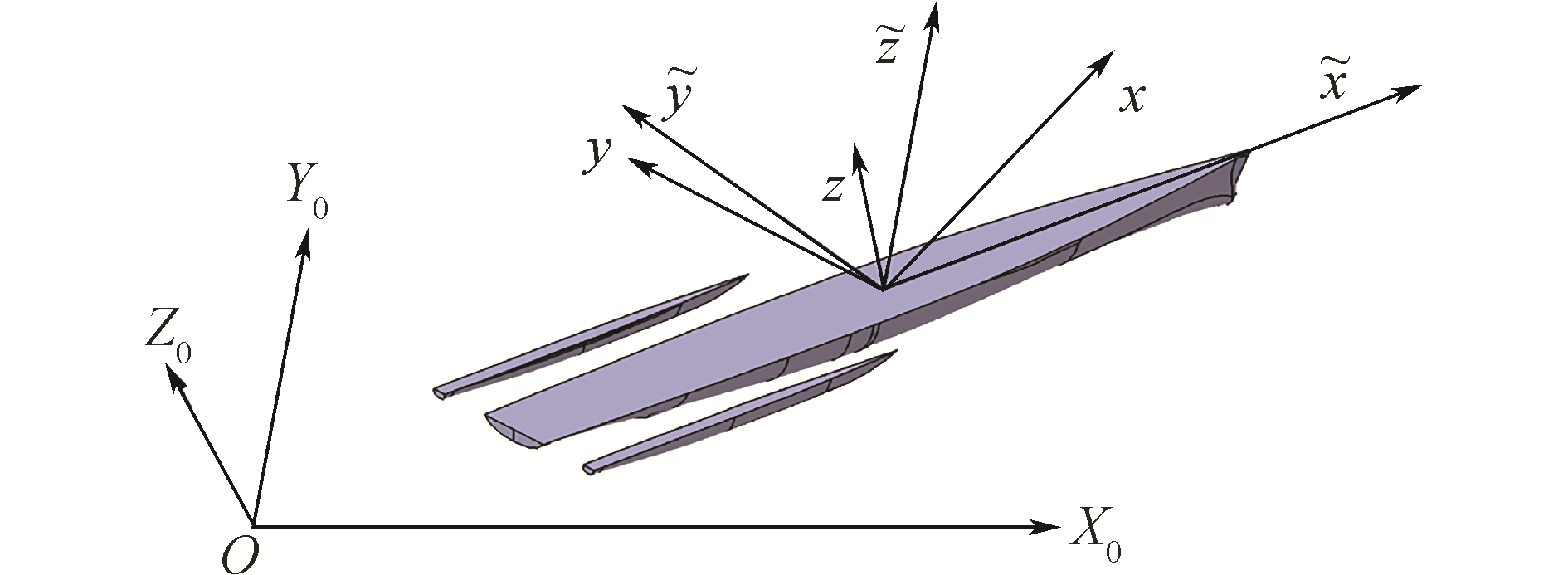Download: 图 1 坐标系示意 Fig. 1 Coordinate system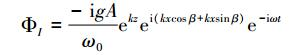(1)

 \begin{aligned} \phi(x, y, z)=& \phi_{I}(x, y, z)+\phi_{D}(x, y, z)+\\ & \sum\limits_{j=1}^{6} \dot{\zeta}_{j} \phi_{j}(x, y, z) \end{aligned} (2)

 $\begin{array}{l} [L]{\nabla ^2}{\phi _j}(x, y, z) = 0\\ [F]\left\{ {\begin{array}{*{20}{l}} { - {\omega ^2}{\phi _j} + g\frac{{\partial {\phi _j}}}{{\partial z}} = 0, }&{自由面{F_1}}\\ {g\frac{{\partial {\phi _j}}}{{\partial z}} - \left( {{\omega ^2} - i\upsilon \omega } \right){\phi _j} = 0, }&{自由面{F_2}} \end{array}} \right.\\ [S]\left\{ {\begin{array}{*{20}{l}} {{{\left. {\frac{{\partial {\phi _j}}}{{\partial n}}} \right|}_{S0}} = {n_j}}\\ {\frac{{\partial {\phi _7}}}{{\partial n}} = - \frac{{\partial {\phi _I}}}{{\partial n}}} \end{array}} \right.\\ [B]\mathop {\lim }\limits_{z \to - \infty } \nabla {\phi _j} = 0\\ [R]\mathop {\lim }\limits_{R \to \infty } \sqrt R \left( {\frac{{\partial {\phi _j}}}{{\partial R}} - ik{\phi _j}} \right) = 0 \end{array}$ (3)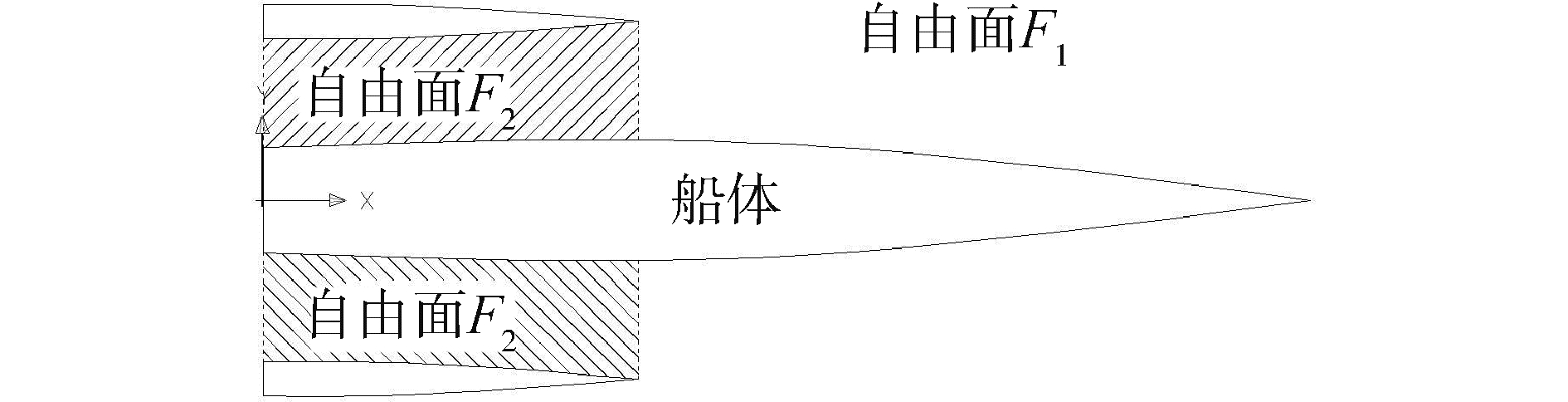Download: 图 2 自由面划分示意 Fig. 2 Division of the free surface

 $\phi_{j}(P)=\iint\limits_{S_{+}+F_{2}} \sigma(Q) G(P, Q) \mathrm{d} s$ (4)

 $\left\{ {\begin{array}{*{20}{l}} {2\pi \sigma (P) + \frac{{{\text{i}}\upsilon \omega }}{g}\iint\limits_{{F_2} + {S_0}} \sigma (Q)G(P, Q){\text{d}}s = 0, } \\ {\;\;\;P \in {F_2}} \\ {2\pi \sigma (P) + \iint\limits_{{F_2} + {S_0}} \sigma (Q)\frac{{\partial G(P, Q)}}{{\partial n}}{\text{d}}s = \frac{{\partial {\phi _j}(P)}}{{\partial n}}, } \\ {\;\;\;P \in {S_0}} \end{array}} \right.$ (5)

 $F_{i}=\rho \iint\limits_{s}\left(\mathrm{i} \omega+U \frac{\partial}{\partial x}\right)\left(\phi_{I}(x, y, z)+\phi_{D}(x, y, z) n_{i}\right) \mathrm{d} S$ (6)

 $\begin{array}{c}{\sum\limits_{j=1}^{6} D_{i j} \zeta_{j}=-\rho \iint\limits_{s} \sum\limits_{j=1}^{6}\left(\mathrm{i} \omega+U \frac{\partial}{\partial x}\right) \zeta_{j} \phi_{j}(x, y, z) n_{j} \mathrm{d} S=} \\ {\sum\limits_{j=1}^{6}\left(\omega^{2} A_{i j}+\mathrm{i} \omega B_{i j}\right) \zeta_{j}}\end{array}$ (7)

 $\sum\limits_{j = 1}^6 {\left[ { - {\omega ^2}\left( {M + {A_{ij}}} \right) - {\rm{i}}\omega {B_{ij}} + {C_{ij}}} \right]} {\zeta _j} = {F_i}$ (8)

2 三体船伪共振特性分析表 1 三体船主尺度 Table 1 Main particulars of a trimaran
2.1 船体水动力模型网格收敛性分析Download: 图 3 三体船水动力模型与试验模型 Fig. 3 Hydrodynamic model and test modle of the trimaran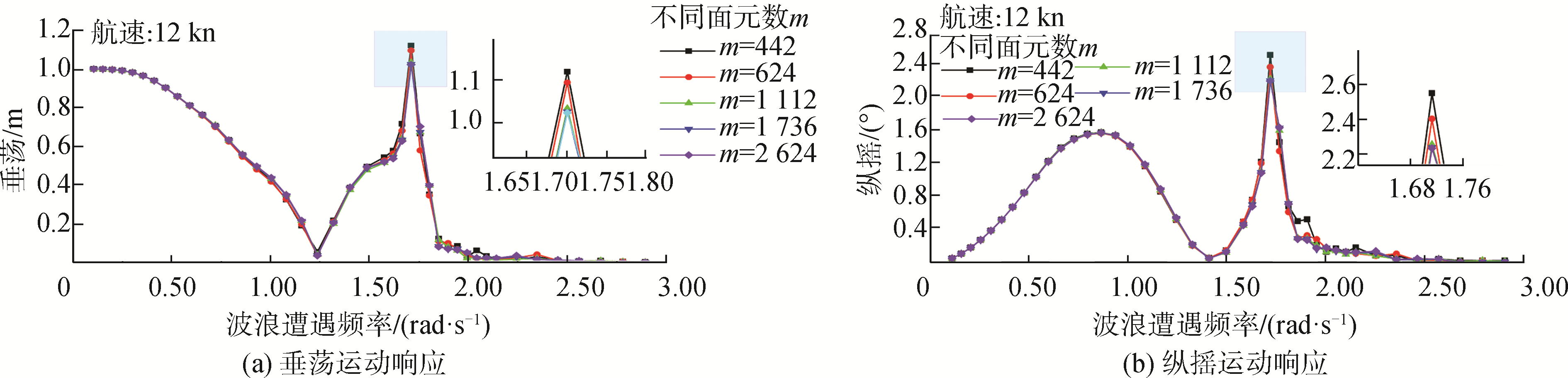Download: 图 4 垂荡、纵摇运动随不同面元数变化 Fig. 4 Heave and pitch variation with different grids
2.2 三体船运动与载荷响应伪共振特性分析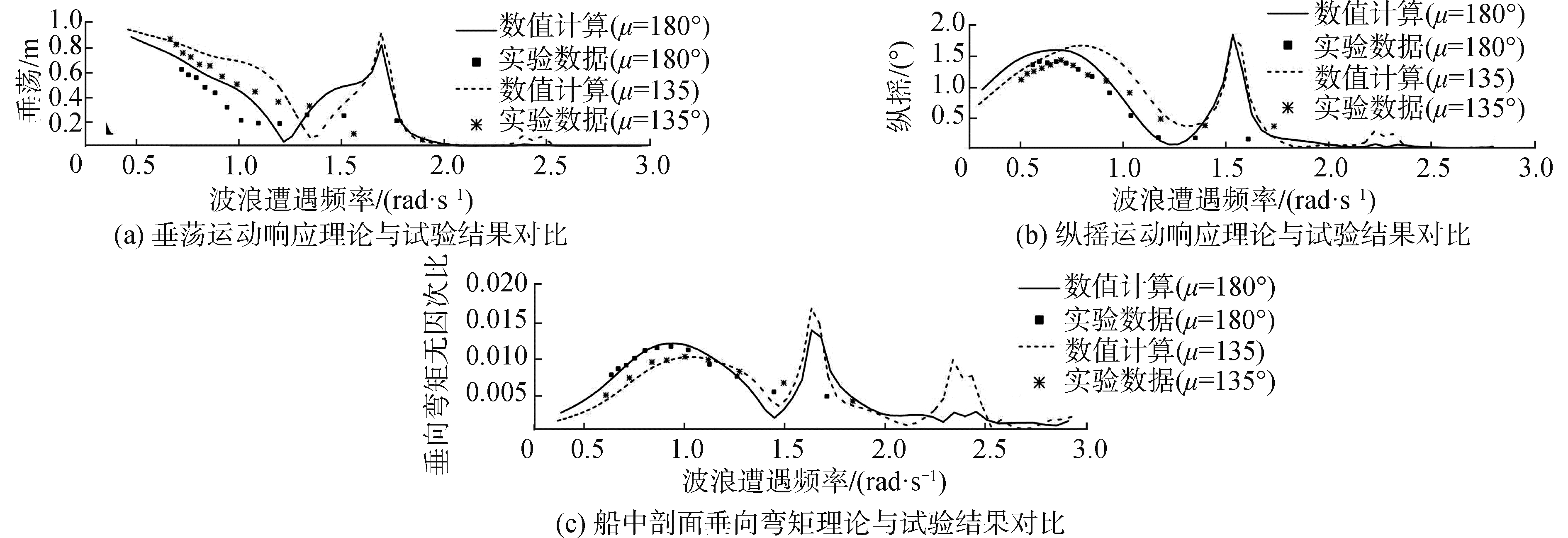Download: 图 5 垂荡、纵摇运动和垂向弯矩理论与试验结果(12 kn) Fig. 5 Theoretical and experimental results of heave, pitch and VBM (12 kn)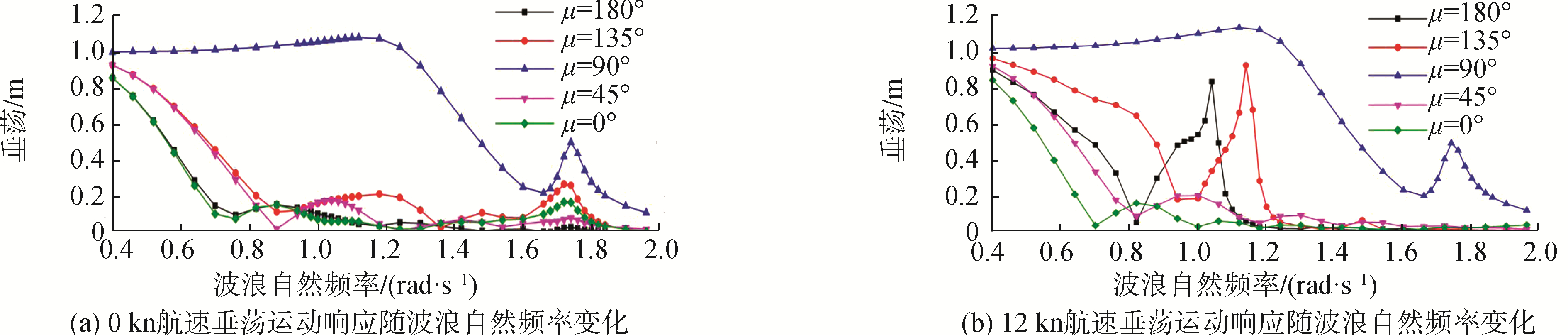Download: 图 6 0、12 kn航速时各浪向下垂荡运动 Fig. 6 Heave in different directions at 0 and 12 knots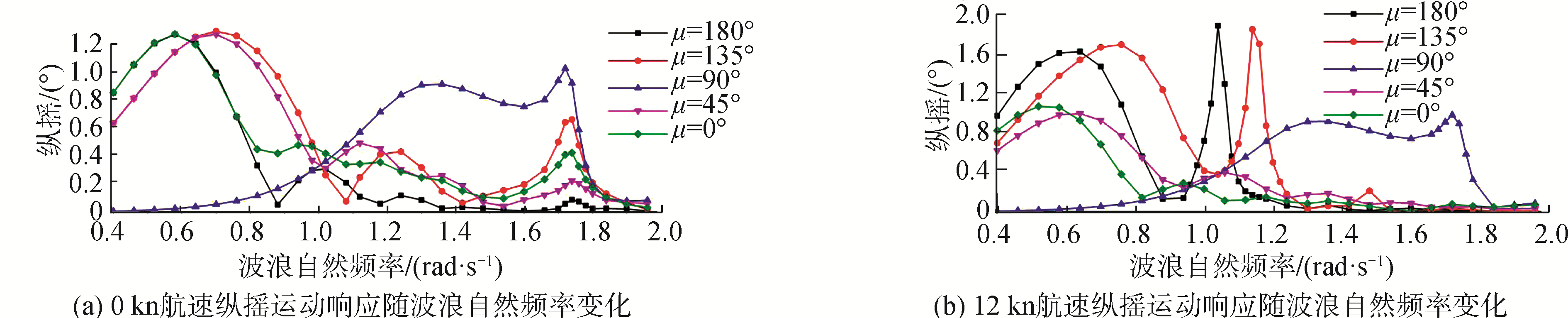Download: 图 7 0、12 kn航速时各浪向下纵摇运动 Fig. 7 Pitch in different directions at 0 and 12 knots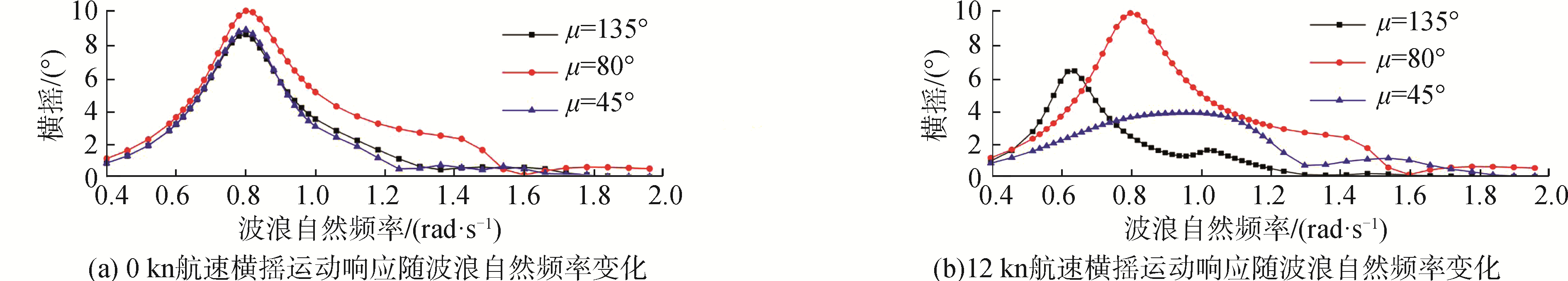Download: 图 8 0、12 kn航速时各浪向下横摇运动 Fig. 8 Roll in different directions at 0 and 12 knots

2.3 片体布置位置对伪共振的影响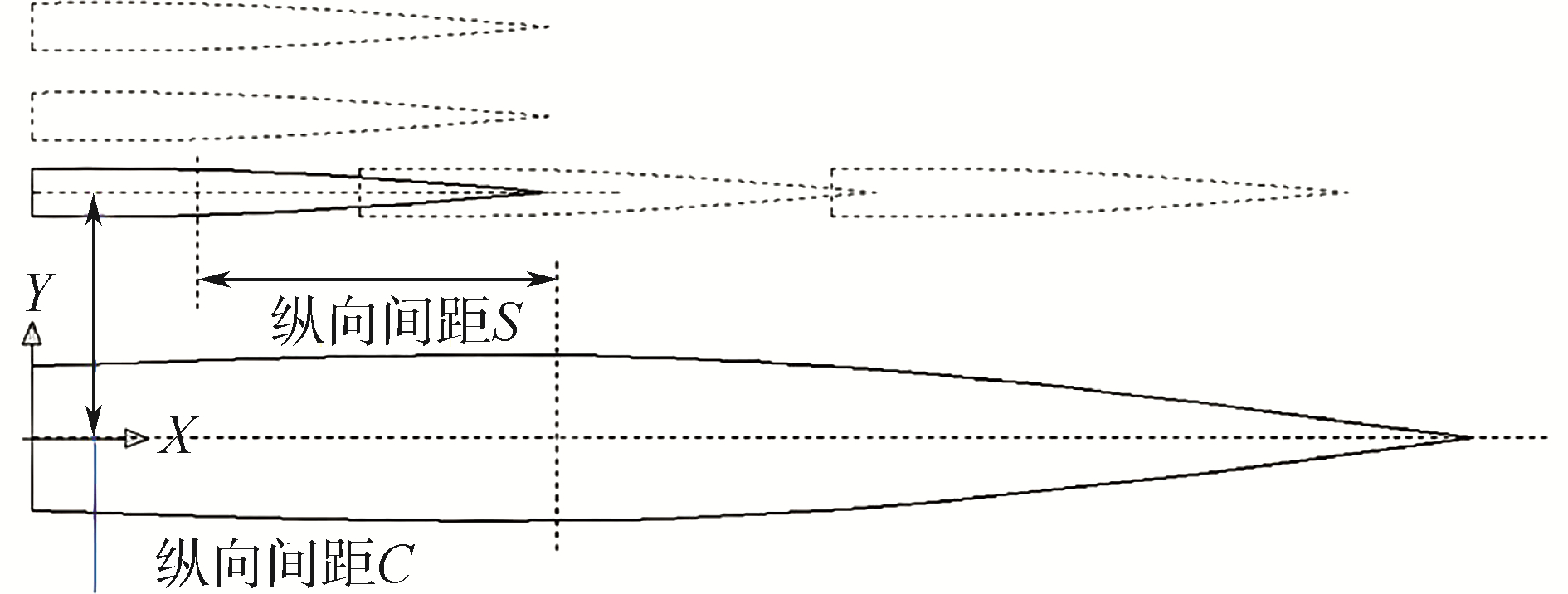Download: 图 9 三体船片体的不同布置位置 Fig. 9 Different layouts of side-hulls of the trimaran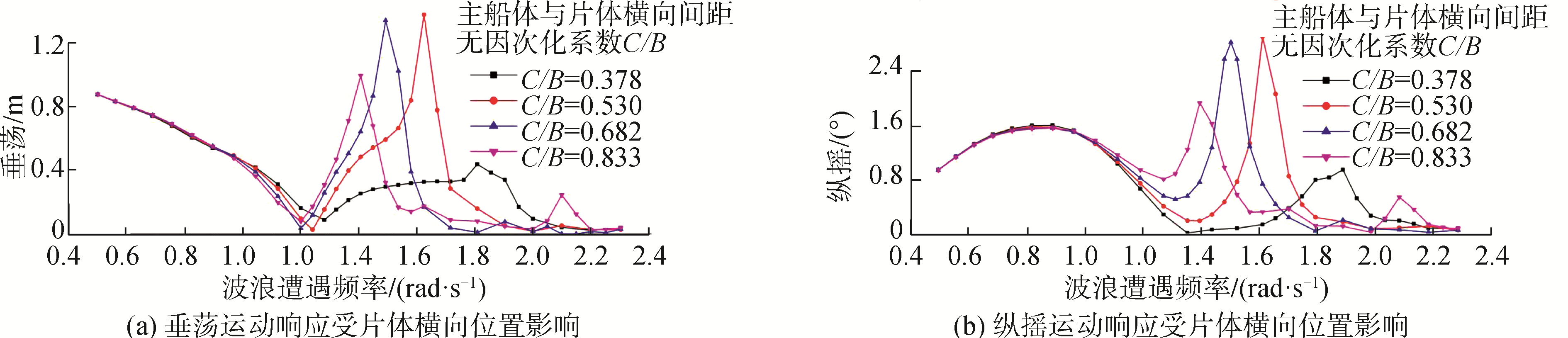Download: 图 10 垂荡和纵摇运动受片体横向位置的影响(12 kn) Fig. 10 Heave and pitch with different transversely position of side-hulls (12 kn)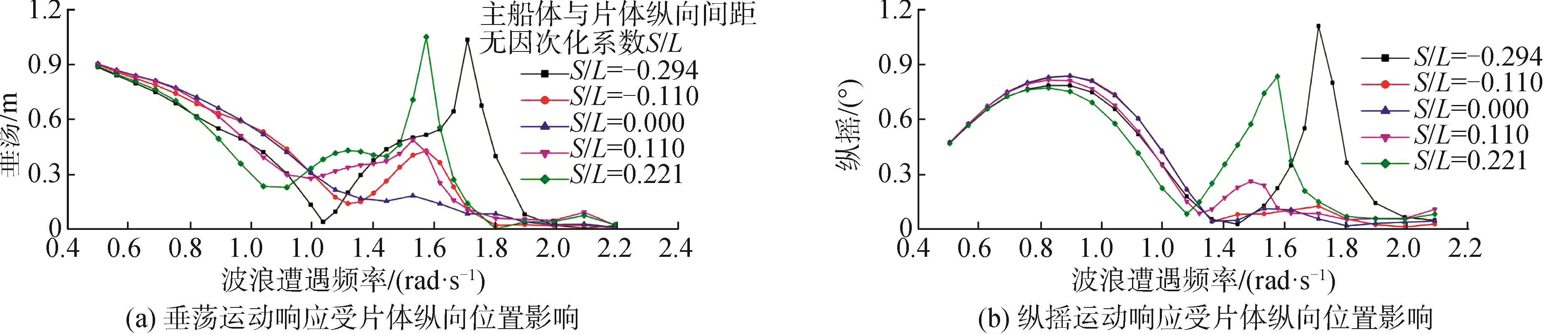Download: 图 11 垂荡和纵摇运动受片体纵向位置的影响(12 kn) Fig. 11 Heave and pitch with different longitudinally position of side-hulls (12 kn)
2.4 辐射与绕射波面特性分析Download: 图 12 自由面上辐射波和绕射波波形 Fig. 12 Wave profile of radiation and diffraction wave on free surface of

3 三体船运动与载荷响应的伪共振修正Download: 图 13 添加不同粘性阻尼后三体船运动响应以及兴波图 Fig. 13 Motion response and wave profile of trimaran after adding different viscous-coefficients

4 结论

1) 对于本文所研究的伪共振问题，航速效应对伪共振的峰值大小有很大影响；在有航速情况下，伪共振的出现浪向角趋于迎浪到横浪之间；三体船的船型布置是影响伪共振的峰值频率变化的主要因素。

2) 伪共振的发生主要是来自纵摇运动与辐射波的耦合作用，而垂荡运动响应上出现伪共振更多的是受到纵摇运动的影响。

3) 拓展的积分方程方法能够有效的用于解决伪共振问题且对于其他频率段的计算结果影响不大，根据本文计算经验，最佳粘性系数一般取为0.1~0.5。

  CHEN Jingpu, ZHU Dexiang, HE Shulong. Research on numerical prediction method for wavemaking resistance of catamaran/trimaran[J]. Journal of ship mechanics, 2006, 10(2): 23-29. (0)  李培勇, 裘泳铭, 顾敏童, 等. 超细长三体船阻力计算研究[J]. 船舶工程, 2002(2): 10-12. LI Peiyong, QIU Yongming, GU Minting, et al. Resistance analysis and calculation of trimaran with super-slender hulls[J]. Ship engineering, 2002(2): 10-12. DOI:10.3969/j.issn.1000-6982.2002.02.002 (0)  陈康, 黄德波. CFD技术在三体船阻力性能研究中的应用[J]. 哈尔滨工程大学学报, 2006, 27(3): 362-366. CHEN Kang, HUANG Debo. Application of CFD technology to the research of resistance performance of trimaran[J]. Journal of Harbin Engineering University, 2006, 27(3): 362-366. DOI:10.3969/j.issn.1006-7043.2006.03.010 (0)  ZHA Ruosi, YE Haixuan, SHEN Zhirong, et al. Numerical computations of resistance of high speed catamaran in calm water[J]. Journal of hydrodynamics, 2014, 26(6): 930-938. DOI:10.1016/S1001-6058(14)60102-5 (0)  WEI Yu, CHEN Xujun, WU Guanghuai, et al. A fast numerical method for trimaran wave resistance prediction[J]. Ocean engineering, 2015, 107: 70-84. DOI:10.1016/j.oceaneng.2015.07.008 (0)  李培勇, 冯铁城, 裘泳铭. 三体船横摇运动[J]. 中国造船, 2003, 44(1): 24-30. LI Peiyong, FENG Tiecheng, QIU Yongming. Investigation of trimaran roll motion characteristics[J]. Shipbuilding of China, 2003, 44(1): 24-30. DOI:10.3969/j.issn.1000-4882.2003.01.004 (0)  LEWANDOWSKI E M. Multi-vessel seakeeping computations with linear potential theory[J]. Ocean engineering, 2008, 35(11/12): 1121-1131. (0)  CASTIGLIONE T, STERN F, BOVA S, et al. Numerical investigation of the seakeeping behavior of a catamaran advancing in regular head waves[J]. Ocean engineering, 2011, 38(16): 1806-1822. DOI:10.1016/j.oceaneng.2011.09.003 (0)  FANG M C, CHEN T Y. A parametric study of wave loads on trimaran ships traveling in waves[J]. Ocean engineering, 2008, 35(8/9): 749-762. (0)  WANG Xueliang, HU Jiajun, GU Xuelian, et al. Comparative studies of the transverse structure design wave loads for a trimaran by model tests and rule calculations[J]. Journal of ship mechanics, 2011, 15(3): 269-275. (0)  XU Min, ZHANG Shilian. A numerical study on side hull optimization for trimaran[J]. Journal of hydrodynamics, 2011, 23(2): 265-272. DOI:10.1016/S1001-6058(10)60112-6 (0)  WU Guanghuai, SHEN Qing, CHEN Xujun, et al. Influence of the distance between floating bodies on hydrodynamic coefficients of floating multi-body system[J]. The ocean engineering, 2003, 21(4): 29-34. (0)  朱仁传, 朱海荣, 缪国平. 具有小间隙的多浮体系统水动力共振现象[J]. 上海交通大学学报, 2008, 42(8): 1238-1242. ZHU Renchuan, ZHU Hairong, LIAO Guopin. Influences on hydrodynamics of multiple floating structures with small gap in between[J]. Journal of Shanghai Jiao Tong University, 2008, 42(8): 1238-1242. DOI:10.3321/j.issn:1006-2467.2008.08.005 (0)  缪国平, 刘应中, 李谊乐, 等. 切片理论应用于双体船运动计算时的伪共振问题[J]. 中国造船, 1997(2): 32-38. LIAO Guopin, LIU Yingzhong, LI Yile, et al. On false resonance in application of strip theory to motion estimation for catamarans[J]. Shipbuilding of China, 1997(2): 32-38. (0)  NEWMAN J N. Double-precision evaluation of the oscillatory source potential[J]. Journal of ship research, 1984, 28(3): 151-154. (0)  缪国平, 刘应中. 关于不规则频率的注记[J]. 水动力学研究与进展, 1985(1): 71-81. LIAO Guoping, LIU Yingzhong. Notes on irregular frequencies[J]. Chinese journal of hydrodynamics, 1985(1): 71-81. (0)  OHMATSU S. On the irregular frequencies in the theory of oscillating bodies in a free surface[R]. Papers of Ship Research Institute, Tokyo, Japan: Ship Research Institute, 1975. (0)